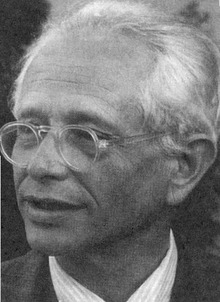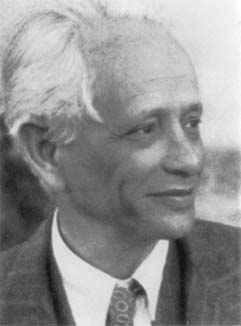# on Geometry, Topology, Dynamics, and Groups

### Academic year 2010 - 2011

 Date Speaker Title — click for abstract (if available) September 8, 2010 Christopher Cashen University of Utah Splitting Line Patterns in Free Groups I will talk about splitting a free group relative to a line pattern. September 22, 2010 Domingo Toledo University of Utah Cubic surfaces and representations of SL(2) It is a classical fact, going back to Fricke and Klein, that the relative representation variety of the fundamental group of the quadruply punctured sphere with fixed traces A, B. C. D at the punctures is an affine cubic surface. Since cubic surfaces depend on 4 parameters it is natural question, observed by several authors, whether every cubic surface can be obtained this way. The purpose of this talk is to present a proof of this fact. This is joint work with Bill Goldman. October 20, 2010 Nat Smale University of Utah Cohomology and Hodge Theory at a Fixed Scale for Metric Spaces For a compact metric space (even as simple as a finite set of points in Euclidean space) there may appear to be nontrivial homology classes at certain fixed scales. I will discuss some recent results on the development of a cohomology theory at a fixed scale for compact metric spaces carrying a Borel measure. For metric spaces that satisfy certain conditions, a corresponding Hodge theory holds. In the special case of Riemannian manifolds at small scales, the corresponding spaces of harmonic functions are isomorphic to the classical ones (and thus the DeRham cohomology). January 31, 2011 Juan Souto University of Michigan Homomorphisms between mapping class groups Suppose that $$X$$ and $$Y$$ are surfaces of finite topological type with genus $$g_X\geq 6$$ and $$g_Y\leq 2g_X-1$$. We describe all homomorphisms $$Map(X)\to Map(Y)$$ between the associated mapping class groups. As a consequence we prove that, if $$X$$ and $$Y$$ have finite analytic type as Riemann surfaces, every non-constant holomorphic map $$M(X)\to M(Y)$$ between the corresponding moduli spaces is a forgetful map. This is join work with Javier Aramayona. February 9, 2011 Richard Hain Duke University Topology and Arithmetic February 16, 2011 Tom Church University of Chicago Representation theory and homological stability Homological stability is a remarkable phenomenon where for certain sequences $$X_n$$ of groups or spaces -- for example $$\mathrm{SL}(n,Z)$$, the braid group $$B_n$$, or the moduli space $$M_n$$ of genus $$n$$ curves -- it turns out that the homology groups $$H_i(X_n)$$ do not depend on $$n$$ once $$n$$ is large enough. But for many natural analogous sequences, from pure braid groups to congruence groups to Torelli groups, homological stability fails horribly. In these cases the rank of $$H_i(X_n)$$ blows up to infinity, and in the latter two cases almost nothing is known about $$H_i(X_n)$$; indeed it's possible there is no nice "closed form" for the answers. While doing some homology computations for the Torelli group, we found what looked like the shadow of an overarching pattern. In order to explain it and to formulate a specific conjecture, we came up with the notion of "representation stability" for a sequence of representations of groups. This makes it possible to meaningfully talk about "the stable homology of the pure braid group" or "the stable homology of the Torelli group" even though the homology never stabilizes. This work is joint with Benson Farb. In this talk I will explain our broad picture and give two major applications. One is a surprisingly strong connection between representation stability for certain configuration spaces and arithmetic statistics for varieties over finite fields, joint with Jordan Ellenberg and Benson Farb. The other is representation stability for the homology of the configuration space of $$n$$ distinct points on a manifold $$M$$. February 25, 2011 Anna Lenzhen University of Rennes 1 — March 9, 2011 Ruth Charney Brandeis University Divergence in right-angled Artin groups The divergence, $$div(\alpha,r)$$, of a geodesic $$\alpha$$ measures the length of the shortest path between two points on $$\alpha$$ that stays outside the ball of radius $$r$$ about their midpoint. We give a group theoretic criterion for determining when a geodesic in a right-angled Artin group $$G$$ has super-linear divergence and show that this divergence is at most quadratic. We use this to describe the structure of the asymptotic cone of $$G$$ and to get a new proof that every non-abelian subgroup of $$G$$ has an infinite dimensional space of quasimorphisms. (Joint work with Jason Behrstock.) April 18, 2011 Anne Thomas University of Sydney Infinite generation of non-cocompact lattices on right-angled buildings Let $$\Gamma$$ be a non-cocompact lattice on a right-angled building $$X$$. Examples of such $$X$$ include products of trees, or Bourdon's building $$I_{p,q}$$, which has apartments hyperbolic planes tesselated by right-angled $$p$$-gons and all vertex links the complete bipartite graph $$K_{q,q}$$. We prove that if $$\Gamma$$ has a strict fundamental domain then $$\Gamma$$ is not finitely generated. The proof uses a topological criterion for finite generation and the separation properties of subcomplexes of $$X$$ called tree-walls. This is joint work with Kevin Wortman. April 27, 2011 Mladen Bestvina University of Utah On the complex of free factors I will give an outline of a proof of the theorem of Masur-Minsky that the curve complex is hyperbolic. The set of nontrivial free factors, up to conjugacy, of a free group of rank n forms the set of vertices of the complex of free factors. I will then discuss hyperbolicity of this complex.

Current seminar          Archive of past talks
Max Dehn Seminar is organized by Mladen Bestvina, Ken Bromberg, Patrick Reynolds,
Jing Tao, Domingo Toledo, and Kevin Wortman.

This web page is maintained by Patrick Reynolds and Jing Tao.